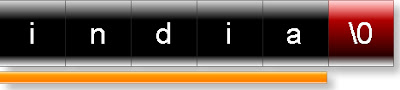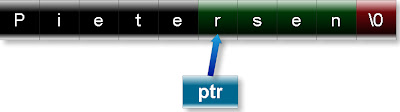## INDEX

### c strlen

strlen is function of c which has been defined inside the library string.h. Prototype of strlen function is:
size_t strlen(const char * str);
Explanation of prototype:
Return type: Return type of strlen function is number of character in the str excluding null character.
Example:
(1)What will be output if you will execute following c code?
#include "string.h"
void main(){
size_t t;
t=strlen("india");
printf("%d",t);
}
Output:
5
Explanation: Strlen function will return number of characters in the string “India” excluding null character as shown in the following figure:Parameter: Its parameter is constant character pointer i.e. string constant of c language.
Example:
(2)What will be output if you will execute following c code?
#include "string.h"
void main(){
int count;
char const *str="Pietersen";
char const *ptr=str;
ptr+=5;
count=strlen(ptr);
printf("%d ",count);
}
Output:
4
Good conceptual questions on strlen function:
(3)What will be output if you will execute following c code?
#include "string.h"
void main(){
int i,j;
i=strlen("taxation system");
j=sizeof("taxation system");
printf("strlen= %d , sizeof= %d ",i,j);
}
Output:
strlen= 15 , sizeof= 16
Explanation: strlen function returns number of character in string excluding null character while sizeof keyword returns size of string including null character.
(4) What will be output if you will execute following c code?
#include "string.h"
void main(){
int x;
x=strlen("\h\1\n\1");
printf("Number of character : %d ",x);
}
Output:
Number of character : 4
Explanation: In c character ‘\’ enjoys special meaning. In this example two characters pair like \h,\1 etc., \ character convert into as single octal character constant.
(5) What will be output if you will execute following c code?
#include "string.h"
void main(){
int num;
num=strlen("\123");
printf("%d ",num);
}
Output:
1
Explanation: character \ converts the character ‘\123’ as a single octal character constant.
(6) What will be output if you will execute following c code?
#include "string.h"
void main(){
int size;
size=strlen("Carala\0baby");
printf("size : %d ",size);
}
Output:
size : 6
Explanation: strlen function returns the number of characters in the string up to null character. In this example character ‘\0’ is null character.
(7) What will be output if you will execute following c code?
#include "string.h"
void main(){
int size;
size=strlen("Paramananda""jha");
printf("%d ",size);
}
Output:
14
(8) What will be output if you will execute following c code?
#include "string.h"
void main(){
int count;
char const *str="Pietersen";
char const *ptr=str;
ptr+=5;
count=strlen(ptr);
printf("%d ",count);
}
Output:
4
Explanation: After incrimination character pointer ptr is pointing to 6th character of string str i.e. r. So strlen function return number of characters in the string “rsen”.(9) What will be output if you will execute following c code?
#include "string.h"
void main(){
int count;
count=strlen("Shane Warne"+8);
printf("%d ",count);
}
Output:
3
(10) What will be output if you will execute following c code?
#include "string.h"
void main(){
int num;
num=strlen("\x4f\x0fc");
printf("%d ",num);
}
Output:
2
Explanation: Character ‘\x’ represents hexadecimal character constantan. In the above question, string has only two hexadecimal character constant.
(11) What will be output if you will execute following c code?
#include "string.h"
void main(){
int num;
num=strlen(NULL);
printf("%d ",num);
}
Output:
0
(12) What will be output if you will execute following c code?
#include "string.h"
void main(){
int num;
num=strlen("NULL");
printf("%d ",num);
}
Output:
4
(13) What will be output if you will execute following c code?
#include "string.h"
void main(){
int size;
size=strlen("\\\\\");
printf("Number of character : %d ",size);
}
Output:
Complier error
Explanation: In c it is necessary to special character ‘\’ are in pair.